# Convert Time to Seconds – Excel & Google Sheets

This tutorial will demonstrate how to convert a time value to seconds in Excel & Google Sheets.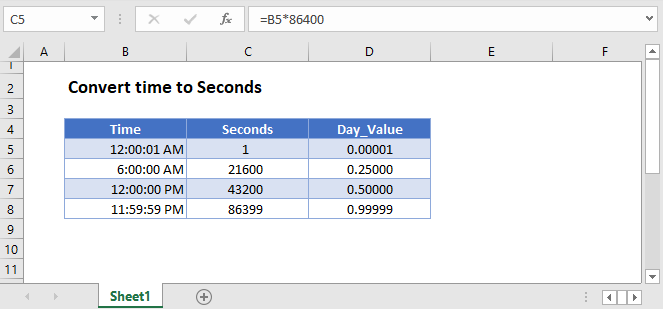## Convert Time to Seconds – Excel

Excel stores time as a decimal value where each 1/24th represents one hour of a day. Understanding this, you can convert a time to seconds by multiplying the time by 86400: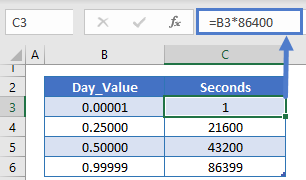Why does this work? Remember that there are 24 hours in a day, 60 minutes in an hour, and 60 seconds in a minute. So there are 24*60*60=86,400 seconds in a day. By multiplying the time by 86,400 you calculate the number of seconds corresponding to the time.

### Format as Number

When you multiply time by 86,400, Excel may format the result as time (ex. h:mm):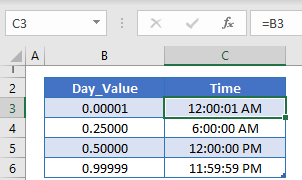To display the result as a regular number, change the formatting to General: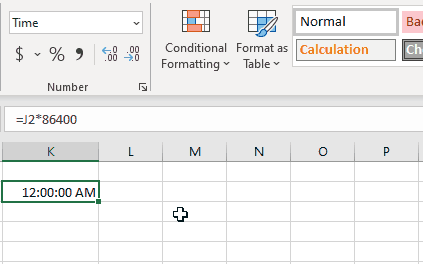## Convert Time to Seconds in Google Sheets

All of the above examples work exactly the same in Google Sheets as in Excel.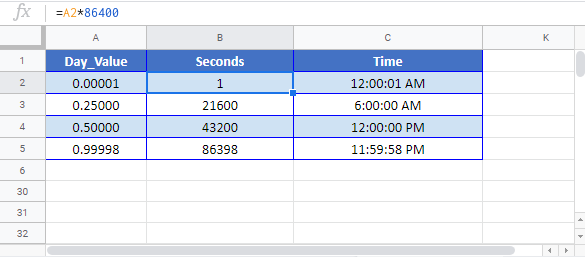### Excel Practice Worksheet

Practice Excel functions and formulas with our 100% free practice worksheets!